Courses

# Test: Level 2: Coordination Chemistry - 2

## 20 Questions MCQ Test Chemistry Class 12 | Test: Level 2: Coordination Chemistry - 2

Description
This mock test of Test: Level 2: Coordination Chemistry - 2 for JEE helps you for every JEE entrance exam. This contains 20 Multiple Choice Questions for JEE Test: Level 2: Coordination Chemistry - 2 (mcq) to study with solutions a complete question bank. The solved questions answers in this Test: Level 2: Coordination Chemistry - 2 quiz give you a good mix of easy questions and tough questions. JEE students definitely take this Test: Level 2: Coordination Chemistry - 2 exercise for a better result in the exam. You can find other Test: Level 2: Coordination Chemistry - 2 extra questions, long questions & short questions for JEE on EduRev as well by searching above.
QUESTION: 1

### Which of the following species is not expected to be a ligand?

Solution:

The correct answer is option B
Complexes are formed when ligands donate a pair of electrons to metals. In ammonia, N atom has one lone pair of electrons. Nitrogen donates this lone pair of electrons to protons to form ammonium ion. So NH4+​ ion does not have a lone pair of electrons which it can donate to central metal ions. Hence it cannot behave as a ligand.

QUESTION: 2

### A complex cation is formed by Pt(in some oxidation state) with ligands (in proper number so that coordination number of Pt becomes six). Which of the following can be its correct IUPAC name?

Solution:

(A) [Pt(en)(SCN)2 (NH3)2]0 ; it is not ion
(B) [Pt(en)(SCN)2 (NH3)2]2+ ; it should not be platinate
(C) correct name
(D) [Pt(en)2(SCN)2(NH3)2]2+ ; its co-ordination number = 8

QUESTION: 3

### Which of the following is not correctly matched?

Solution:

Sodium(ethylenediaminetetraacetato)chromate(II) and Na2​[Cr(CH3​COO)4​(en)] are not correctly matched.
Sodium(ethylenediaminetetraacetato)chromate(II) is the name of the coordiation compound having formula Na2​[Cr(edta)]
The name of the compound Na2​[Cr(CH3​COO)4​(en)] is sodium tetraacetato(ethylenediamine)chromate(II).

QUESTION: 4

The EAN of metal atoms in [Fe(CO2)(NO+)2] and Co2(CO8) respectively are:

Solution:

Oxidation state of iron in [Fe-2(CO0)2 (NO+)2] = x+2(0) + 2 = 0; x= -2

So EAN = 28 =8=36

Oxidation state of cobalt in Co2(CO)8 = 2x + 8 (-8) = x=0

So Ean = 27 + 1+ 8 = 36

QUESTION: 5

Match the complex ions listed in column-I with the characteristics listed in column-II using the codes given below.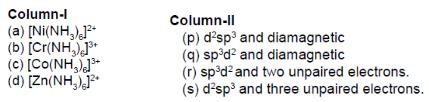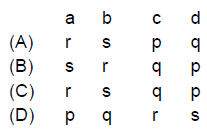Solution: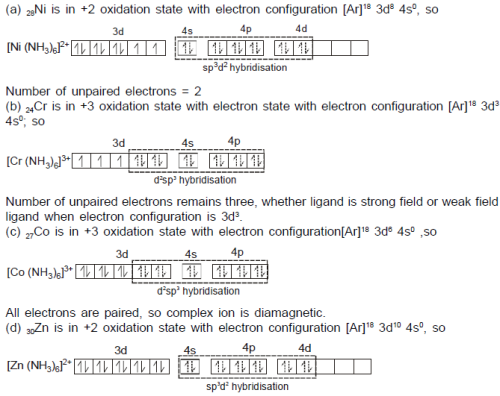QUESTION: 6

Which of the following statements is correct?

Note : NO is three electrons donor

Solution: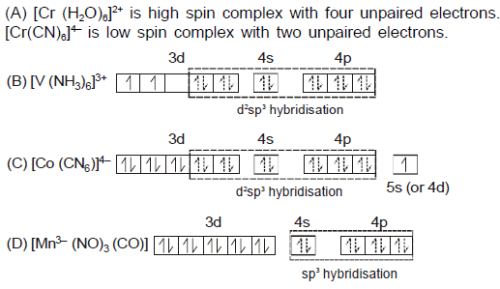QUESTION: 7

All the following complex ions are found to be paramagnetic
P : [FeF6]3- ; Q : [COF6] 3- ; R : [V(H2O)6] 3+ ; S : [Ti(H2O)6]3+
The correct order of their paramagnetic moment (spin only) is

Solution: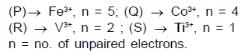QUESTION: 8

Among [NiCl2 (PPh3)2], [Ni(HDMG)2] and [Ni(CO)4] complexes the hybridisation statesof Ni atom are respectively.

Solution: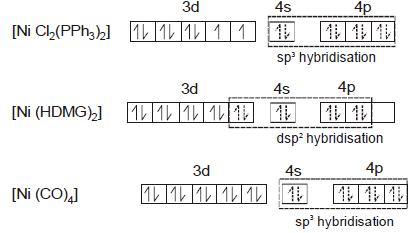QUESTION: 9

Amongst [Co(ox)3]3- , [CoF6]3- and [Co(NH3)6]3+

Solution: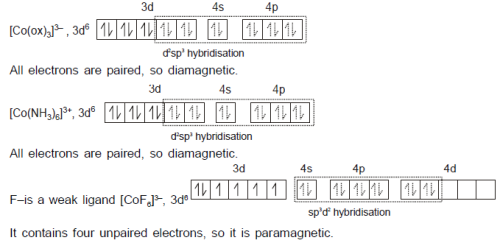QUESTION: 10

All the following complexes show decrease in their weights when placed in a magnetic
balance then the group of complexes having tetrahedral geometry is:
(i) Ni(CO)4

(ii) K[AgF4]

(iii) Na2[Zn(CN)4]

(iv) K2[PlCl4]

( v )[RhCl(PPh3)3]

Solution: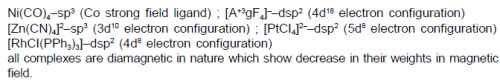QUESTION: 11

The ‘brown ring’ formed at the junction of two layers in the test of nitrate is due to the formation of a complex ion, [Fe(H2O)5NO]2+. Which of the following statements are correct for this complex [u = 3.87 B.M.)
(i) Oxidation state of Fe is +1 and NO exists as NO+.
(ii) The complex ion is in octahedral geometry as attained by sp3d2 hybridisation.
(iii) The complex is paramagnetic and has three unpaired electrons due to transfer of  electron from NO to Fe2+.
(iv) The complex is in octahedral geometry as attained by d2sp3 hybridisation.
(v) The brown colour of the complex is attributed to d-d transition of electron.

Solution: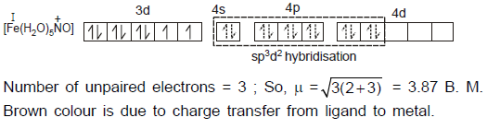QUESTION: 12

Which of the following statements is most likely to be incorrect?

Solution: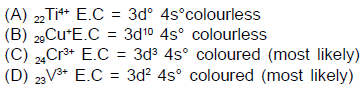QUESTION: 13

Identify the correct order of wavelength of light absorbed for the following complex ions.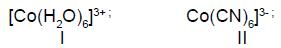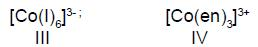Solution: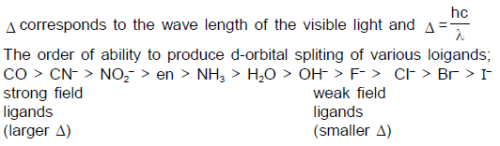QUESTION: 14

The possible geometric isomers shown by [Co(en)Br2Cl2]- is:

Solution: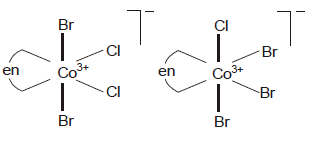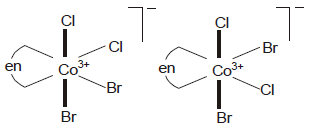QUESTION: 15

The total number of isomers of the complex, [Co(NH3)4(NO2) 2(NO3) complex is :

Solution: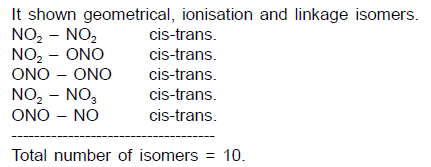QUESTION: 16

Which of the following has both face-mer and optical isomers?

Solution: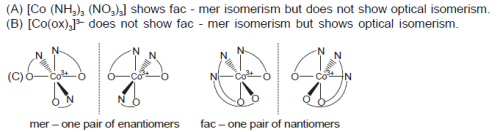QUESTION: 17

Statement–1 : In complex [Cr(NH3)4 BrCl]Cl, the ‘spin only’ magnetic moment is close to 1.73 B.M.
Statement–2 : All known mononuclear complexes of chromium(III), irrespective of the strength of the ligand field, must have three unpaired electrons.

Solution: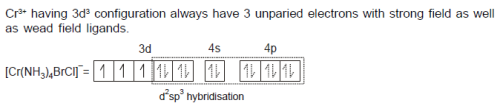QUESTION: 18

Statement–1 : The magnitude of for a given ligand increases as the charge on he metal ion increases.
Statement–2 : NH3 is a weak field ligand towards Co2+ but acts as a strong field ligand towards Co3+.

Solution:

As metal ion charge increases the ligands are drawn closer to the metal ion because
of its increased charge density. As the ligands move closer, they cause greater splitting of tghe d-orbital, there by producing a large Δ value.

QUESTION: 19

Statement–1 : tris (ethane-1, 2 – diamine)nickel(II) chloride is homoleptic outer orbital complex
Statement–2 : The geometry of Ni in tris (ethane-1, 2 – diamine)nickel(II) chloride is trigonal bipyramidal

Solution: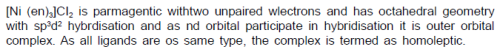QUESTION: 20

Statement–1 : [Ni (H2O)6]2+ is green but a solution of [Ni(CN)4]2- is colourless.
Statement–2 : Energy difference between d levels (i.e. ) for H2 O complex (paramagnetic) is in the visible region and that for the cyano complex (diamagnetic) is in the UV region

Solution:

Cyanide is stronger ligand than water. It causes much higher crystal field splitting. Energy difference between d levels (i.e.)for [Ni(H2O)6]2+ complex (paramagnetic) is in the visible region and that for the [Ni(CN4)]2 complex (diamagnetic) is in the UV region.
Hence, [Ni(H2O)6]2+ forms green color solution whereas [Ni(CN4)]2 forms colorless solution.